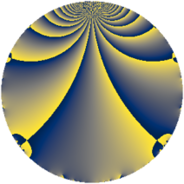Properties

 Label 539.2.aLevel $539$ Weight $2$ Character orbit 539.a Rep. character $\chi_{539}(1,\cdot)$ Character field $\Q$ Dimension $34$ Newform subspaces $12$ Sturm bound $112$ Trace bound $3$

Related objects

Defining parameters

 Level: $$N$$ $$=$$ $$539 = 7^{2} \cdot 11$$ Weight: $$k$$ $$=$$ $$2$$ Character orbit: $$[\chi]$$ $$=$$ 539.a (trivial) Character field: $$\Q$$ Newform subspaces: $$12$$ Sturm bound: $$112$$ Trace bound: $$3$$ Distinguishing $$T_p$$: $$2$$, $$3$$

Dimensions

The following table gives the dimensions of various subspaces of $$M_{2}(\Gamma_0(539))$$.

Total New Old
Modular forms 64 34 30
Cusp forms 49 34 15
Eisenstein series 15 0 15

The following table gives the dimensions of the cuspidal new subspaces with specified eigenvalues for the Atkin-Lehner operators and the Fricke involution.

$$7$$$$11$$FrickeDim.
$$+$$$$+$$$$+$$$$5$$
$$+$$$$-$$$$-$$$$13$$
$$-$$$$+$$$$-$$$$11$$
$$-$$$$-$$$$+$$$$5$$
Plus space$$+$$$$10$$
Minus space$$-$$$$24$$

Trace form

 $$34 q + q^{2} - q^{3} + 37 q^{4} + 3 q^{5} + 6 q^{6} + 3 q^{8} + 31 q^{9} + O(q^{10})$$ $$34 q + q^{2} - q^{3} + 37 q^{4} + 3 q^{5} + 6 q^{6} + 3 q^{8} + 31 q^{9} + 4 q^{10} + 2 q^{11} - 6 q^{12} - 2 q^{13} - 5 q^{15} + 39 q^{16} + 4 q^{17} + 7 q^{18} + 12 q^{20} + q^{22} - q^{23} + 16 q^{24} + 43 q^{25} - 6 q^{26} - 7 q^{27} - 10 q^{29} - 46 q^{30} - 13 q^{31} - 33 q^{32} - q^{33} + 2 q^{34} + 15 q^{36} + 33 q^{37} - 20 q^{38} + 16 q^{39} - 6 q^{40} + 18 q^{41} - 6 q^{43} + q^{44} + 28 q^{45} - 26 q^{46} - 16 q^{47} + 8 q^{48} + 5 q^{50} - 22 q^{51} - 26 q^{52} + 44 q^{53} + 34 q^{54} - q^{55} - 8 q^{57} + 6 q^{58} - 3 q^{59} - 34 q^{60} + 10 q^{61} - 6 q^{62} + 71 q^{64} + 28 q^{65} - 14 q^{66} - 3 q^{67} + 6 q^{68} + 13 q^{69} + q^{71} - 49 q^{72} - 2 q^{73} + 36 q^{74} - 16 q^{75} - 20 q^{76} - 80 q^{78} + 22 q^{79} - 10 q^{80} - 22 q^{81} - 10 q^{82} - 22 q^{83} + 66 q^{85} - 60 q^{86} + 60 q^{87} - 9 q^{88} + 5 q^{89} - 42 q^{90} - 62 q^{92} + 17 q^{93} + 36 q^{94} - 40 q^{95} - 32 q^{96} + 15 q^{97} + 11 q^{99} + O(q^{100})$$

Decomposition of $$S_{2}^{\mathrm{new}}(\Gamma_0(539))$$ into newform subspaces

Label Dim. $$A$$ Field CM Traces A-L signs $q$-expansion
$a_{2}$ $a_{3}$ $a_{5}$ $a_{7}$ 7 11
539.2.a.a $1$ $4.304$ $$\Q$$ None $$-2$$ $$1$$ $$-1$$ $$0$$ $-$ $-$ $$q-2q^{2}+q^{3}+2q^{4}-q^{5}-2q^{6}-2q^{9}+\cdots$$
539.2.a.b $1$ $4.304$ $$\Q$$ None $$0$$ $$-1$$ $$-3$$ $$0$$ $-$ $+$ $$q-q^{3}-2q^{4}-3q^{5}-2q^{9}-q^{11}+\cdots$$
539.2.a.c $1$ $4.304$ $$\Q$$ None $$0$$ $$3$$ $$1$$ $$0$$ $-$ $+$ $$q+3q^{3}-2q^{4}+q^{5}+6q^{9}-q^{11}+\cdots$$
539.2.a.d $1$ $4.304$ $$\Q$$ None $$1$$ $$-2$$ $$2$$ $$0$$ $-$ $-$ $$q+q^{2}-2q^{3}-q^{4}+2q^{5}-2q^{6}-3q^{8}+\cdots$$
539.2.a.e $2$ $4.304$ $$\Q(\sqrt{2})$$ None $$-2$$ $$0$$ $$0$$ $$0$$ $+$ $+$ $$q-q^{2}+\beta q^{3}-q^{4}-\beta q^{5}-\beta q^{6}+3q^{8}+\cdots$$
539.2.a.f $2$ $4.304$ $$\Q(\sqrt{5})$$ None $$0$$ $$-2$$ $$4$$ $$0$$ $-$ $+$ $$q-\beta q^{2}+(-1-\beta )q^{3}+3q^{4}+2q^{5}+\cdots$$
539.2.a.g $3$ $4.304$ $$\Q(\zeta_{18})^+$$ None $$0$$ $$-3$$ $$-6$$ $$0$$ $-$ $-$ $$q+\beta _{1}q^{2}+(-1+\beta _{1}-\beta _{2})q^{3}+\beta _{2}q^{4}+\cdots$$
539.2.a.h $3$ $4.304$ 3.3.257.1 None $$0$$ $$-1$$ $$-2$$ $$0$$ $+$ $+$ $$q+\beta _{2}q^{2}-\beta _{1}q^{3}+(1+\beta _{1}-\beta _{2})q^{4}+\cdots$$
539.2.a.i $3$ $4.304$ 3.3.257.1 None $$0$$ $$1$$ $$2$$ $$0$$ $-$ $+$ $$q+\beta _{2}q^{2}+\beta _{1}q^{3}+(1+\beta _{1}-\beta _{2})q^{4}+\cdots$$
539.2.a.j $3$ $4.304$ $$\Q(\zeta_{18})^+$$ None $$0$$ $$3$$ $$6$$ $$0$$ $+$ $-$ $$q+\beta _{1}q^{2}+(1-\beta _{1}+\beta _{2})q^{3}+\beta _{2}q^{4}+\cdots$$
539.2.a.k $4$ $4.304$ 4.4.9248.1 None $$2$$ $$0$$ $$0$$ $$0$$ $-$ $+$ $$q-\beta _{3}q^{2}+\beta _{2}q^{3}+(2-\beta _{3})q^{4}+(\beta _{1}+\cdots)q^{5}+\cdots$$
539.2.a.l $10$ $4.304$ $$\mathbb{Q}[x]/(x^{10} - \cdots)$$ None $$2$$ $$0$$ $$0$$ $$0$$ $+$ $-$ $$q+\beta _{5}q^{2}+\beta _{1}q^{3}+(2+\beta _{9})q^{4}+\beta _{7}q^{5}+\cdots$$

Decomposition of $$S_{2}^{\mathrm{old}}(\Gamma_0(539))$$ into lower level spaces

$$S_{2}^{\mathrm{old}}(\Gamma_0(539)) \cong$$ $$S_{2}^{\mathrm{new}}(\Gamma_0(11))$$$$^{\oplus 3}$$$$\oplus$$$$S_{2}^{\mathrm{new}}(\Gamma_0(49))$$$$^{\oplus 2}$$$$\oplus$$$$S_{2}^{\mathrm{new}}(\Gamma_0(77))$$$$^{\oplus 2}$$